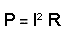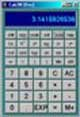# Power & Energy

• After studying this section, you should be able to:
• Carry out calculations involving power, voltage, current and resistance.
• • using appropriate units and sub-units.
• Differentiate between power and energy in electrical circuits.

## Power in Resistors

When a current flows through a resistor, electrical energy is converted into HEAT energy. The heat generated in the components of a circuit, all of which possess at least some resistance, is dissipated into the air around the components. The rate at which the heat is dissipated is called POWER, given the letter P and measured in units of Watts (W).

The amount of power dissipated can be worked out using any two of the quantities used in Ohms law calculations. Remember, as with any formula the BASIC QUANTITIES must be used in the formula, i.e. VOLTS, OHMS and AMPERES, (not milli, Meg etc).

To find the power P using V and ITo find the power P using V and RTo find the power P using I and RBefore starting, think about these few tips, they will make the problems easier if followed carefully.

1. Work out the answers using pencil and paper; otherwise it is easy to get mixed up half way through and end up with the wrong answer.

2. Of course the answer is not just a number, it will be a certain number of Watts (or multiple or sub units of Watts). Don't forget to show the correct unit (e.g. W or mW etc.) as well as the number or the answer is meaningless.

3. Convert all sub units such as mV or kΩ to Watts when you put them into the appropriate formula. A slip up here will give really stupid answers, thousands of times too big or too small.

4. Although the structure of these power formulae seems very similar to the Ohms Law formulae, there is a subtle difference - they contain some squared terms (I2 and V2). Be very careful if using the triangle trick to transpose these formulae. If you need to relate power to resistance, then either I or V must be squared (multiplied by itself). However you can construct a triangle to fit either of the formulae to give R, as shown below.Don't forget to download our ‘Maths Tips’ booklet, which shows you how to use your calculator with exponents and engineering notation to deal with those sub-units and get the right answer every time.

Not got a scientific calculator? The ‘Maths Tips’ booklet explains what you need (and what you don't need so you don't spend your money unnecessarily). If you don't want to buy a scientific calculator, you can always pick up a free one on the net. PC users can try Calc98 from www.calculator.org/download.html. Whichever calculator you choose, read the instructions to become familiar with the working methods you should use as these do vary from calculator to calculator.

It is important to be aware of the effect of power dissipation in components, the greater the power, the more heat must be dissipated by the component. This generally means that components dissipating large amounts of power get hot, also they will be considerably larger in size than low power types. If a component is required to dissipate more power than it is designed to, it will not be able to get rid of the heat generated fast enough. Its temperature will rise and the overheating may cause complete failure of the component and possibly damage to other components and the printed circuit board (PCB) itself. As a precaution, large power resistors are often mounted clear of the PCB by using longer lead out wires encased in ceramic sleeves. High power wirewound resistors may even be encased in a metal heat sink and bolted to a large metal area such as the equipment case, to get rid of unwanted heat. Examples of high power resistors are shown on the Resistor Construction page.

Components such as resistors have a particular power rating quoted by the manufacturer (in Watts or milli Watts). This rating (parameter) must be checked when replacing a component so that no over rating will occur. This is an important safety consideration when servicing electronic equipment.

### TIP

The heat generated by high power resistors is a major cause of early failure in many circuits. Either the resistor itself fails by going "open circuit", especially in wire wound resistors. In carbon composition resistors, overheating over a long period can cause the value to change. This may increase in high resistance types, or more dangerously reduce (allowing an increased current flow) in low value types. The increase in current flow caused by this reduction in resistance only accelerates the process and eventually the resistor (and sometimes other associated components) burns up!

### Energy in Resistors

If a certain amount of power is dissipated for a given time, then ENERGY is dissipated. Energy (power x time) is measured in Joules and by including time (t) in the power formulae, the energy dissipated by a component or circuit can be calculated.

Energy dissipated = Pt or VIt or V2t/R or even I2Rt Joules

Note that in formulae for energy, quantities such as power, time, resistance, current and voltage must be converted to their basic units, e.g. Watts, seconds, Ohms, Amperes, Volts etc. No sub units or multiple units! As described in the ‘Maths Tips’ booklet.

All of the above units are part of an integrated system of internationally standardised units; the S.I. (Système International d´Unités) System. This system sets out the basic units for any electrical, mechanical and physical property and their relations to each other. It also includes the standard form of multiples and sub multiples described in the ‘Maths Tips’ booklet.

Top of Page.>